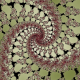## Fractals with non-Integer powers

I mess around with fractals a lot, and sometimes stumble on some interesting things. Recently I came across some odd jagged/discontinuous fractals:This tends to happen in fractals as soon as I involved non-Integer powers.

Why is this happening? To understand this, we need to look at some basic complex analysis.

Recall that a complex number can be interpreted as a vector like$(real, imaginary)$. But we can also think if a vector in terms of direction and magnitude. For our purposes, we will think of vectors as a magnitude and an angle from the positive portion of the real number line (sometimes called the argument,’ we will just call it the angle).

Complex numbers have the interesting property that when we multiply them their magnitudes multiply like normal numbers but their angles add. For example,$-1$ has an angle of$\pi$ so when we multiply two of them we end up with a magnitude of$1$ and an angle of$2\pi$ and thus$1$.

When we raise a value to a real power, the angle is multiplied by that value. We can visualise this as:$z \to z^2$$\implies$It’s fairly clear that for the inverse, for any value, there are two valid answers.$\implies$$z^2\to z$We can also look at it with an alternative visualisation I just cooked up:Notice that the angle of the ray of discontinuity is arbitrary, but its existence is inevitable.

(For more on complex analysis, I recommend Visual Complex Analysis by Tristan Needham (Google Books, Amazon). It’s awesome!)

(Sage users: Here is a generalised version of the function I used to make the above image. It takes a value n and returns a visualisation of the nth root:


def root_visualize(n):
l=[]
for j in range(n*8):
l.append(complex_plot(lambda x: (x*exp(-sqrt(-1)*j*pi/4))^(1/n)*exp(sqrt(-1)*j*pi/n/4),[-5,5],[-5,5]))
print j
return animate(l)
`

Be warned: it is slow)

Now, notice that$z^{1.5}$ is the same as$z\sqrt{z}$. Thus, it has the same discontinuity. That seems like a reasonable answer to “why is this so jagged?”

But it leads to some interesting questions. The intuitive reaction is (for me at least): part of the fractal is missing. You’re cutting apart the nice, smooth mutlifunction and thus are only seeing part of the fractal. Well, at$n$ iterations there$2^n$ possible ways to have cut this hypothetical super-fractal (as I will refer to it).

Let’s look at the Julia super-fractal of$z\to \pm z^{1.5}+0.3$ for four iterations…If you stare at all the possibilities of the forming set you will notice some patterns and what look like reciprocals and other patterns. One may intuitively wish to add them to extract a single fractal, but this isn’t possible since they’d cancel… Perhaps adding the absolute values?

(For more on the formation of fractals, see my previous post.)

Of course, one really should look at a larger number of iterations than I am. There is the small problem of this being$O(n*2^n)$, but it should be easy to parallelize…

So that’s the extent that I’ve explored this to so far. I’d be thrilled to get some feedback. Do you have any thoughts about this? Know of similar things that have been studied? (I’ve never formally studied Chaos Theory and suspect I have lots of holes in my knowledge. Reminder to self: take/audit course on chaos theory next year…)

Tags: , ,

### 7 Responses to “Fractals with non-Integer powers”

1.Munero Says:

Also try the inverse julia plotting to pass through discontinuities and view the whole set.

•christopherolah Says:

This is a very clever idea.

Sadly, I can’t come up with any situations where this solves the problem, since the power is a non-integer greater than one (and thus its reciprocal is a non-integer…). But I like the thought.

2.Munero Says:

With f(z)=(z-c)^(2/3), which is a three-valued function,
by iterating (f(f(…f(f(0))…)), n times), we have 3^n points that show the global shape of the entire set. But the plot is dirty, ald looks like a cloud.

To see -> http://hpics.li/1c09c0b

•christopherolah Says:

Fascinating! Thanks for making that! Now I really want to calculate it 🙂 Combine them using the super norm over the branches… With some GPGPU stuff, it should be practical!

3.Munero Says:

Taking c=-2, this gives Sierpinski-Koch-look Julia sets.
These are more clear when c is far from zero.

•christopherolah Says:

Will do.

By the way, while I’ve been to busy to make a GPGPU implementation, I brute forced it with a pruned binary tree implementation to 18 iterates.Rather surprising. I’m wondering if it has just been too few iterates for it too disconnect, if some branch (an alternating one?) doesn’t result in a disconnected set, or if all the branches result in disconnected sets and they just fit together to be a connected one…

4.Munero Says:

In my opinion, this set is disconected for all branches, and the red area is zero. It can be visible with more iterations.